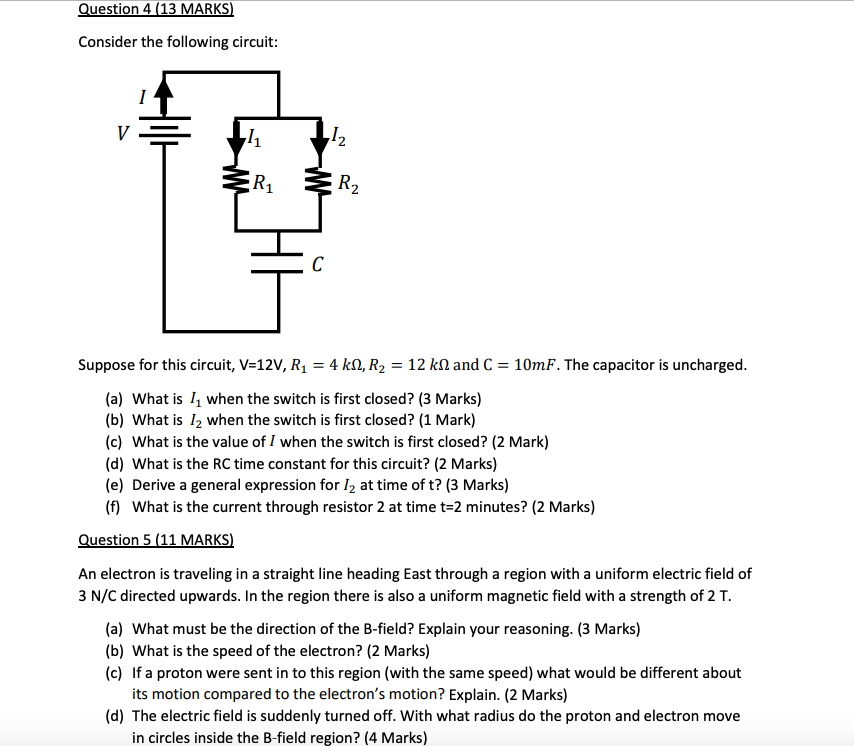Consider the following circuit: 2 1  2 Suppose for this circuit, V-12V, R1-4 kQ, R2-12 kΩ and C-10mF. The  capacitor is uncharged. (a) What is 11 when the switch is first closed?  (3 Marks) (b) What is 12 when the switch is first closed? (1 Mark) (c)  What is the value of I when the switch is first closed? (2 Mark) (d)  What is the RC time constant for this circuit? (2 Marks) (e) Derive a  general expression for /2 at time of t? (3 Marks) (f) What is the  current through resistor 2 at time t 2 minutes? (2 Marks) An electron is  traveling in a straight line heading East through a region with a  uniform electric field of 3 N/C directed upwards. In the region there is  also a uniform magnetic field with a strength of 2 T (a) What must be  the direction of the B-field? Explain your reasoning. (3 Marks) (b) What  is the speed of the electron? (2 Marks) (c) If a proton were sent in to  this region (with the same speed) what would be different about its  motion compared to the electron's motion? Explain. (2 Marks) (d) The  electric field is suddenly turned off. With what radius do the proton  and electron move in circles inside the B-field region? (4 Marks)• Posted: 3 months ago
answer

Purchase the answer to view itSave time and money!
Our teachers already did such homework, use it as a reference!• Not rated

### Consider the following circuit: 2 1 2 Suppose for this circuit, V-12V, R1-4 kQ, R2-12 kΩ and C-10mF. The capacitor is uncharged.

Consider the following circuit: 2 1 2 Suppose for this circuit, V-12V, R1-4 kQ, R2-12 kΩ and C-10mF. The capacitor is uncharged. (a) What is 11 when the switch is first …